# Perform calculation from values of the first table to the values on the second table

Please help! I want to divide values from a certain column of the 1st table to the values on the second table that has a row containing the name of the certain column from the 1st table.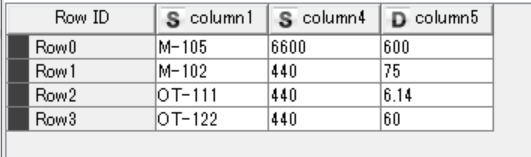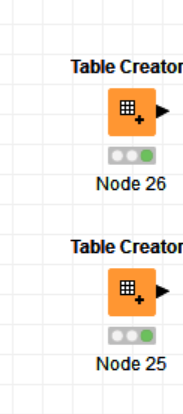Hi ikesh!

Welcome to the forum!

I have a small question about your use case that is not clear to me. For instance, for the name M-105 you would like to divide 350 (Row1) by 6600 (column 4) and 60 (Row 2) by 600 (column 5), and 70 would be not divided by anything? Or would you like to divide each values for M-105 in table 1 by the sum of the values in the row containing M-105 for table 2?

Cheers,
Ana

1 Like

Hi! Thank you for your response. I need to divide the average of values in the column M-105 (table1) with the value on column 5 (table2) i.e. in row 0 of table2. For ex. 1)mean(350,60,70) divide by 600
2) mean(500,440,200) divide by 60

First use M-105, OT-122 so on as column names.
Use Transpose node for the first table

and then Column Aggregator

Then use Joiner to join transpose table with second one on field with values M-105,…

and finally calculate on join resul table using Math Formula node
1 Like

Hi @ikesh,

@izaychik63 already presented a very good solution! I also have an example workflow, you can check it here:

https://hub.knime.com/ana_ved/space/Compute_Division_between_tables

The idea is very similar to what @izaychik63 has proposedHope it helps!

Cheers,
Ana

1 Like

Hi,

Here you are:

2 table division.knwf (19.5 KB)

First calculate the column mean for the first table. Transform the values to flow variables. Divide in Column Expressions with this expression:

`variable(column("column1")) / column("column2")`2 Likes

@armingrudd2 Likes

Hi , your replies are very much appreciated. But there’s no math formula(for multi-column) and column expression on my Knime version. The link on the forum was not found. Also, please see my data below. I already get the mean per day in every column and I need to divide those values by looking up a value from the row on the second table which contained the column name of the first.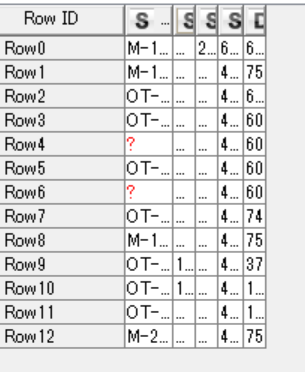Thank you in advance. Good day!

2 Likes

Hi, thank you very much for your continuous support. However, I don’t have admin privilege to be able to download extensions which include math formula(multi-column) and column expression. Is there any other means to come up with the same answer but different solution (not using multi-column math formula, and column expression)?. Moreover, what if table 2 has names which is not exactly the same as column names of the first, but has just characters which contained in the column names of the first table? . Thanks again.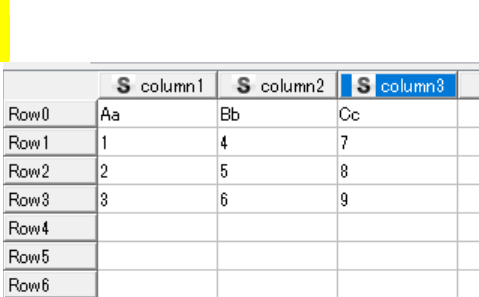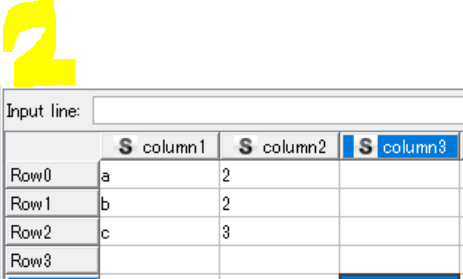Hi there,

Don’t know your company policy but either you should ask for admin rights on this matter or ask someone with admin rights to install extensions on your KNIME instance. Nodes from KNIME extensions are pretty powerful and can help you much in your daily workBr,
Ivan

2 Likes

Are the values of “column1” in the second table unique?
And are they only the first character of the column names of the first table?

I have built a workflow but need to make sure it fits your case.Hi @ikesh!

Would these two columns and tables always have the same order? I.e, the first column in table 1 will always correspond to the first row in table 2? Or the order can change?

Also, do you usually read the data from a excel file or something similar? There is an option there to read the first row as table headers. Maybe that would be useful in case the first row in table 1 is always the columns headers.

Well,

It seems you are busy and cannot answer my questions right now. Just to make sure I won’t miss this topic, I provide the current workflow I have built. This workflow uses the base nodes only .

The only problem here is that how exactly your column headers from the first table become the values of the column1 in the second table. For now I have assumed that it’s the first character and they are unique. If this is not the case please let me know and I will modify the workflow for you again.

2 table division.knwf (43.7 KB)2 Likes

Hi again. Thank you so much for the answers. Now, I would like to use the following table. The same scenario, Averages of the 1st table will be divided to the corresponding values on the second table via character matching. For ex. Average(1st table) / 2nd table. Note: corresponding values. Also, I want to loop on the number of rows, as you notice, there a number of rows, but I will get the average per unique row number(i.e. per day) and then loop. Thank you so much.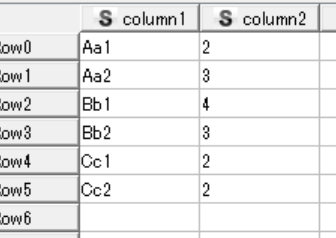• First you have to read the first row in the first table as column header in the reader node you are using.
• Use a GroupBy node and the Day column as the grouping column and then apply the “Mean” aggregation on the other columns. In the “Advanced settings” section select “Keep original names” for column naming.
• Now use a Chunk Loop Start node and pass its output to the Transpose node.
• Join the current table and the second table based on the Row ID form the current table and the column1 from the second table.
• Finally add a Loop End (Column Append).Hi, do you have a sample workflow,? Thank you again.

Here you are:

2_table-div.knwf (333.4 KB)

This workflow is built regarding your last example tables. If you need to manipulate the column names to match the second table values, please check the previous workflow and make your desired changes in the current one.1 Like

Hi there again! After I’ve followed your advice, I am now moving on. Thanks for the effort. And now, I need to format the table so that it will be suitable for analysis. Look for example the attached images. I need to arrive to the final table from a given initial table. Thanks in advance.

Hi @ikesh

I suggest to do a Pivot node twice (on Item and Item2) and then join them together. See this wf, pivot_2.knwf (25.3 KB)gr. Hans

1 Like